Support your 3rd grader by giving him these double digit addition worksheets to practise his fundamental maths skills. Working on these worksheets will help him as he adds triple digit numbers later in the year.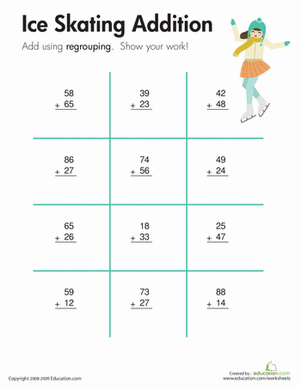### Ice Skating: Two-Digit Addition with Regrouping

Kids solve addition problems with two-digit addends using regrouping on this year four maths worksheet.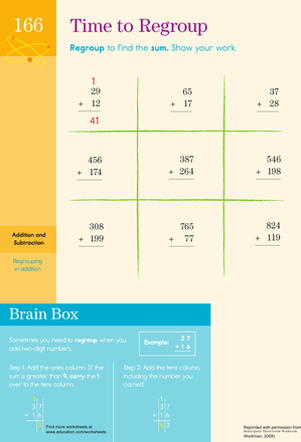Searching for a worksheet that practices addition regrouping? This printable is perfect for practising addition skills like carrying the tens column.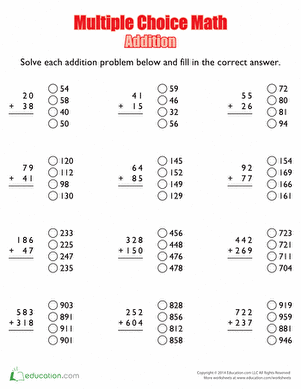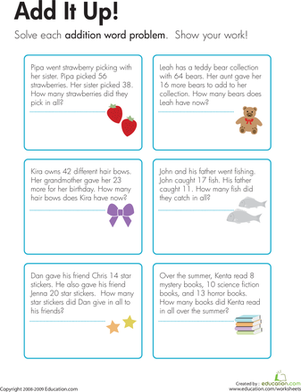Kids use details from the word problems on this year four maths worksheet to construct and solve addition problems with two or three two-digit addends.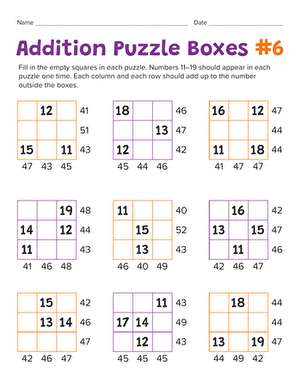Add a fun twist to maths practise with these addition puzzles. Your maths maniac will get an introduction to logic and problem solving.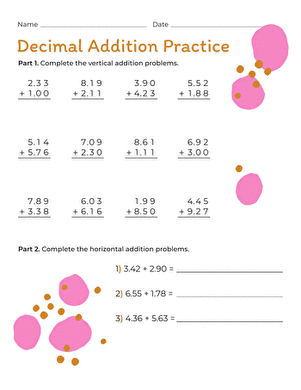Teach your third grader the power of the decimal point. He'll practise decimal addition with this worksheet.

Create new collection

0

### New Collection>

0Items

What could we do to improve Education.com?